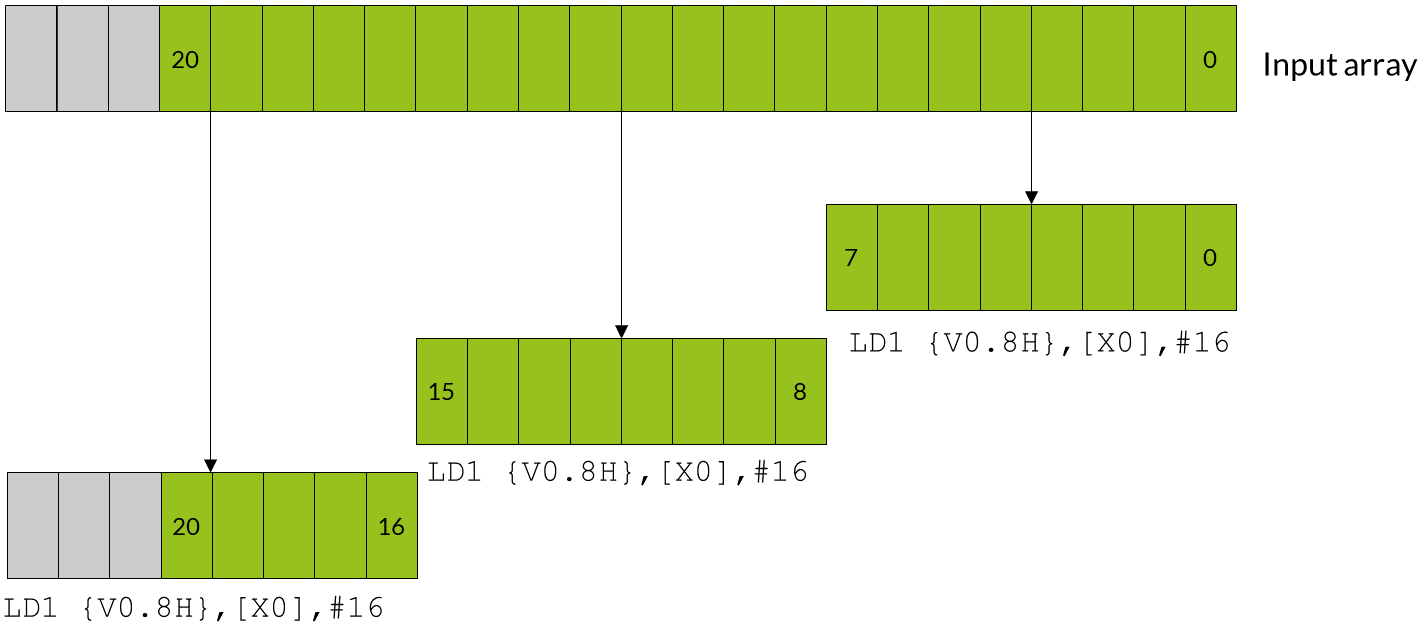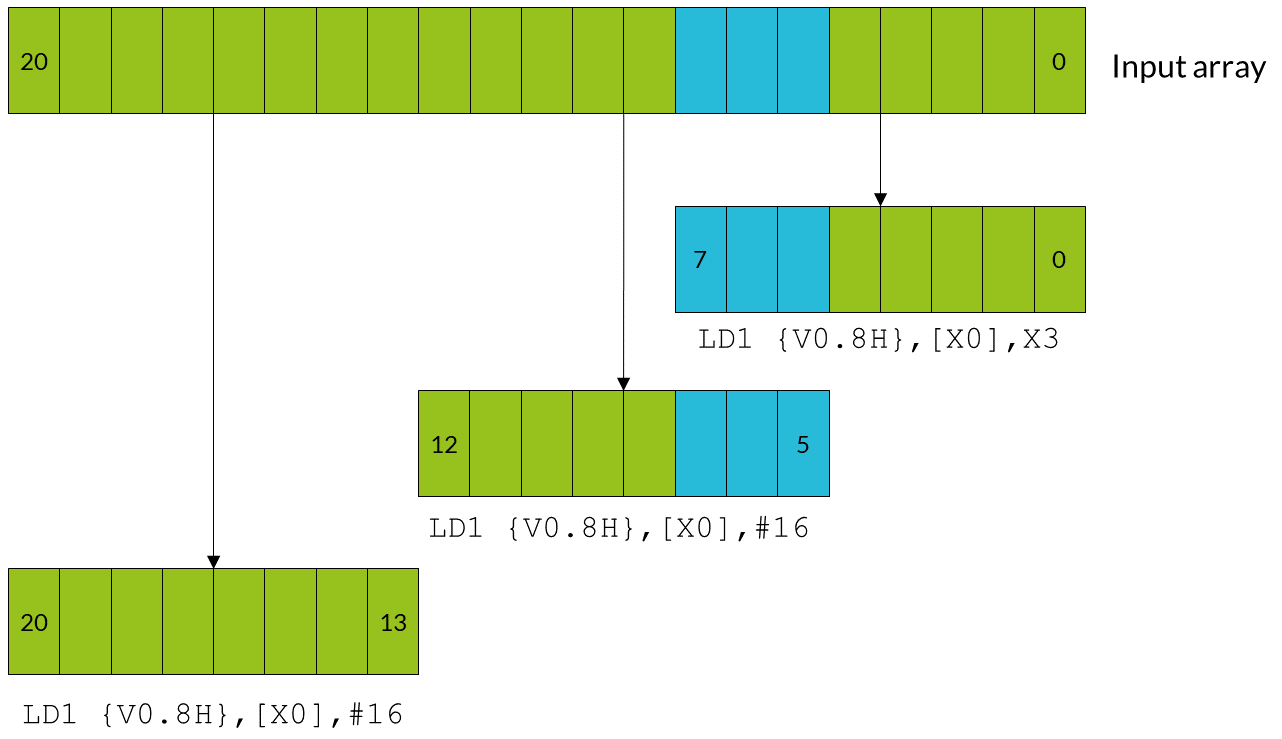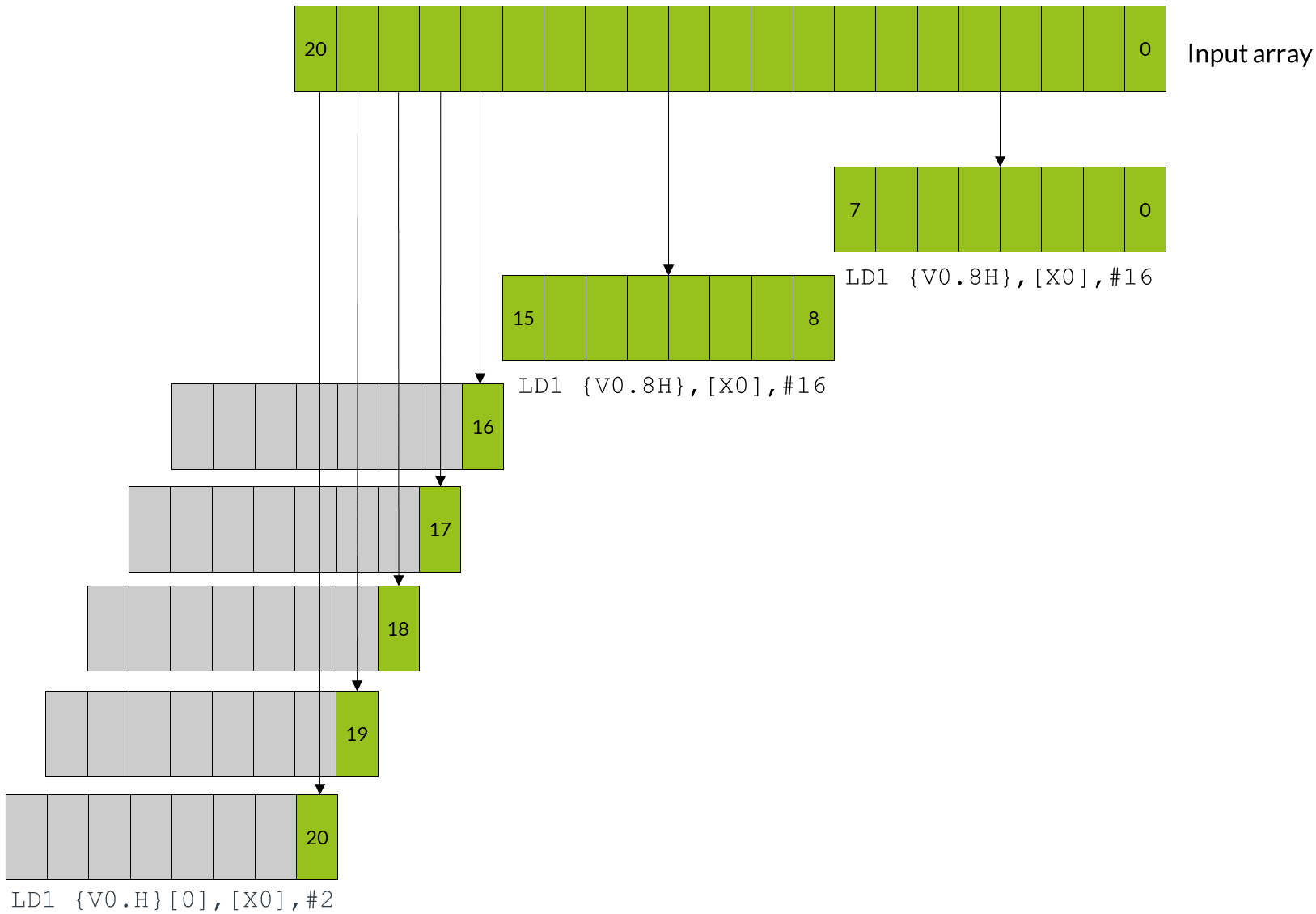## Load and store - leftovers

A common situation when coding for Neon is dealing with input data that is not an exact multiple of the number of lanes in the vector register.

For example, consider an input array that contains 21 data elements, each of which is a 16-bit integer. You want to use Neon to process the data in this array. Neon registers are 128 bits wide, so can process eight lanes of 16-bit data at a time. In two iterations, your Neon code can process 16 (2 x 8) data elements. However, this leaves five leftover data elements to process in the final iteration. These five leftover data elements are not enough to completely fill a Neon register.

There are three approaches that you can take to handle these leftovers. Which method to choose depends on your requirements. The three approaches are as follows, with the fastest approach listed first:

If you can change the size of the arrays, you can increase the length of the array to the next multiple of the vector size using padding elements. This allows you to read and write beyond the end of your data without corrupting adjacent storage.

In our example with 21 data elements, increasing the array size to 24 elements allows the third iteration to complete without potential data corruption.

The following diagram shows how the three iterations load eight data elements into the Neon register. The final iteration loads the three padding elements along with the final five array values:The gray data elements in the diagram represent padding values, and the green data elements are the original 21 array values.

Be careful to choose padding values that do not affect the result of your calculation. For example:

• If you are summing array values, use a paddingvalue of zero.
• If you are finding the minimum value in an array, use a padding value of the maximum value that the data element can contain.

It might not be possible to choose a padding value that does not affect the result of your calculation. For example, when calculating the range of an array of numbers any padding value you choose could affect the result. In these cases, do not use this method.

Note:Allocating larger arrays consumes more memory. The increase could be significant if many short arrays are involved.

The following code shows how you could implement a solution that extends arrays with padding:

```// Function entry point
//    X0 = input array pointer
//    X1 = output array pointer
//    X2 = number of elements in array

process_array:
ADD X2, X2, #7    // Add (vector register lanes - 1) to the array length
LSR X2, X2, #3    // Divide the length of the array by the number of
//   vector register lanes (8) to find the number of
//   iterations required.

loop:
LD1 { V0.8H } , [X0], #16  // Load eight elements from the array pointed to
//   by X0 into V0, and update X0 to point to the
//   next vector
//...
//...                      Process the data for this iteration
//...

ST1 { V0.8H } , [X1], #16  // Write eight elements to the output array, and
//   update X1 to point to next vector

SUBS X2, X2, #1            // Decrement the loop counter and set flags
B.NE loop                  // Branch back if count is not yet zero...
RET                        // ... otherwise return```

## Overlap data elements

If the operation is suitable, leftover elements can be handled by overlapping those elements. Overlapping means processing some of the elements in the array twice.

In the example case, the iterations that use overlap would follow these steps:

1. The first iteration processes elements zero to seven.
2. The second iteration processes elements five to 12.
3. The third and final iteration processes elements 13-20.

Note that elements five to seven,  which are the overlap between the first vector and the second vector, are processed twice.

The following diagram shows how all three iterations load eight data elements into the Neon register, with the first and second iterations operating on overlapping vectors:The blue data elements represent the overlapping elements that are processed twice. The green data elements are the original 21 array values.

You can only use overlaps when the operation applied to the input data does not vary with the number of times that the operation is applied. The technical term is to say that the operation must be idempotent. For example, if you are trying to find the maximum element in an array, you can use overlaps. This is because it does not matter if the maximum value appears more than once. However, if you are summing an array, you cannot use overlaps. This is because the overlapping elements would be counted twice.

Note:The number of elements in the array must fill at least one complete vector.

The following code shows how you could implement a solution that extends arrays with padding:

```// Function entry point
//    X0 = input array pointer
//    X1 = output array pointer
//    X2 = number of elements in array

process_array:
ANDS X3, X2, #7  // Calculate number of elements left over after
//   processing complete vectors using
//   array length & (vector register lanes - 1).

LSL X3, X3, #1   // Multiply leftover elements by 2 to get the required
//   address increment because we are dealing with doubleword data.

BEQ loopsetup    // If the result of the ANDS is zero, the length
//   of the data is an exact multiple of the number
//   of lanes in the vector register, so there is
//   no overlap. Processing can begin.

// Otherwise, handle the first vector separately...
LD1 {V0.8H}, [X0], X3 //  Load the first eight elements from the array,
//  and update the pointer by the required address increment.
//...
//...                     Process the data for this iteration.
//...

ST1 {V0.8H}, [X1], X3  // Write eight elements to the output array, and
//   update the pointer.

// Now set up the vector processing loop
loopsetup:
LSR  X2, X2, #3      // Divide the length of the array by the number of lanes
//   in the vector register (8) to find the number of
//   iterations required.

//  This loop can now operate on exact multiples
//   of the lane number. The first few elements of
//   the first vector overlap with some of those
//   processed earlier.
loop:
LD1 { V0.8H }, [X0], #16    // Load eight elements from the array pointed to
//   by X0 into V0, and update X0 to point to the
//   next vector.
//...
//...                           Process the data for this iteration.
//...

ST1 { V0.8H }, [X1], #16        // Write eight elements to the output array, and
//   update X1 to point to next vector.

SUBS X2, X2, #1                 // Decrement the loop counter and set flags
B.NE loop                       // Branch back if count is not yet zero...
RET                             // ... otherwise return ```

## Process leftovers as single elements

Neon provides load and store instructions that can operate on single elements in a vector. You can use these instructions to load a partial vector that contains one element, operate on that partial vector, and then write the element back to memory.

In the example case, the iterations using single elements would follow these steps:

1. The first two iterations execute as normal, processing elements zero to seven, and eight to 15.
2. The third iteration needs only to process five elements. A separate loop handles these elements, which loads, processes, and stores single elements.

The following diagram shows how the first two iterations operate on full vectors, while the leftover elements are handled individually:This approach is slower than the previous two methods. This is because each leftover element must be loaded, processed, and stored individually.

This approach increases code size. Handling leftovers individually requires two loops, one for the full vectors, and a second loop for the single elements.

Note:Neon single element loads only change the value of the specified lane in the destination element, leaving the rest of the vector intact. If the calculation that you are performing involves instructions that work across a vector, the register must be initialized before loading the first single element. For example, if you were using `ADDV` to sum across the entire vector, initialize the unused lanes to zero.

The following code shows how you could implement a solution that processes leftovers as single elements:

```// Function entry point
//    X0 = input array pointer
//    X1 = output array pointer
//    X2 = number of elements in array

process_array:
LSR X3, X2, #3      // Calculate the number of complete vectors to be
//   processed.

CMP X3, #0
BEQ  singlesetup     // If there are zero complete vectors, branch to
//   the single element handling code.

// Process vector loop.
vectors:
LD1 {V0.8H}, [X0], #16  // Load eight elements from the array and update
//   the pointer by eight doublewords.
//...
//...                   Process the data for this iteration.
//...

ST1 {V0.8H}, [X1], #16  // Write eight elements to the output array, and
//   update the pointer by eight doublewords.
SUBS X3, X3, #1         // Decrement the loop counter, and set flags.

BNE  vectors            // If X3 is not equal to zero, loop.

singlesetup:
ANDS X3, X2, #7         // Calculate the number of single elements to process.
BEQ  exit               // If the number of single elements is zero, branch to exit.

// Process single element loop.
singles:
LD1 {V0.H}, [X0], #2   // Load single element into lane 0, and update the
//   pointer by one doubleword.
//...
//...                     Process the data for this iteration.
//...

ST1 {V0.H}, [X1], #2   // Write the single element in lane zero to the
//   output array, and update the pointer.

SUBS X3, X3, #1           // Decrement the loop counter, and set flags.
BNE  singles              // If X3 is not equal to zero, loop.
exit:
RET```

## Other considerations for leftovers

The three approaches can be refined or adapted to suit your own particular needs as follows:

• Choose when to process leftover elements

You can choose to apply the overlapping and single element techniques at either the start, or the end, of processing an array. The examples in this guide can be adapted to process leftover elements at either end of processing, depending on which is more suitable for your application.